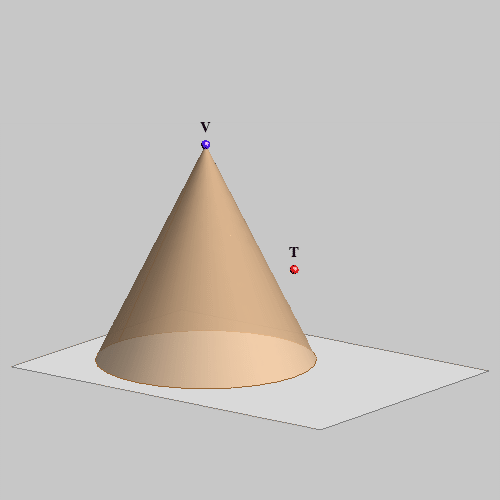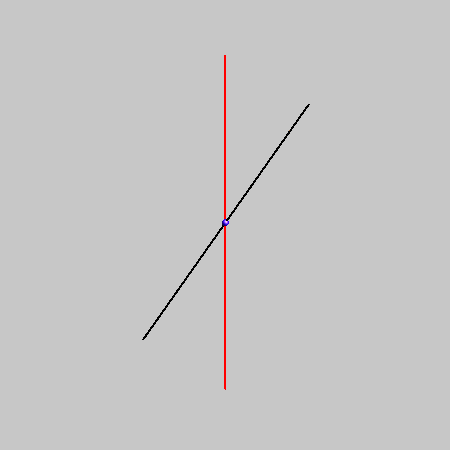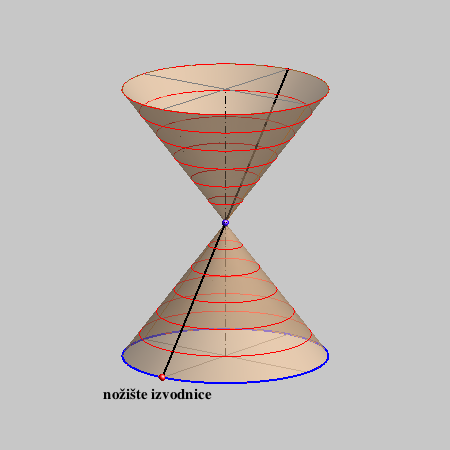## Cones of the 2nd degree

• The 2nd degree cones are algebraic ruled surfaces whose rulings are connecting points of a non-degenerated conic k with a point that doesn't lie in the plane of the conic, called the vertex V.

### Plane intersections of the 2nd degree cones

Every plane intersection of the 2nd degree cone is a conic.

REMINDER: proper conics

REMINDER: degenerated curves

If a conic has a double point, it is degenerated into a pair of different real lines or a pair of conjugate imaginary lines with a real intersection point. Beside these two degenerated conics, there is also a degenerated conic that has infinitely many double points - one real double line.

#### 1. A plane contains the vertex of the cone

If a plane contains the vertex of a cone, then it intersects the cone in two generatrices of the cone, i.e. this plane intersection is a degenerated conic.

A plane through the vertex intersects the curve k in two points, lines connecting these points with the vertex lie in this plane and they are generatrices of the cone. These intersection points can be real and different, real and coincide or conjugate imaginary points - the generatrices can be real and different, coincide or imaginary. Therefore, there are 3 cases of the plane intersection:

• a pair of real intersecting lines,

• one real double line,

• a pair of conjugate imaginary lines that intersect at a real point.

 two real generatrices a double line - a tangent plane two conjugate imaginary lines

Right-click to start the animation.

#### 2. A plane does NOT contain the vertex of the cone

Any plane that does not contain the vertex of a cone intersects the cone in a proper conic.

The type of the conic depends on the points at infinity of this conic. Points of the inetersection curve are intersection points of generatrices of the cone and the plane. Remember that a line intersects a plane at the point at infinity if they are parallel. Therefore, we conclude the following:

A plane intersection with the plane not passing the vertex is

• a hyperbola, if the plane is parallel to two real generatrices,

• a parabola, if the plane is parallel to one real generatrix,

• an ellipse, if the plane is parallel to none of the generatrices.

 HYPERBOLA a plane parallel to two generatrices PARABOLA a plane parallel to one generatrix ELLIPSE a plane is not parallel to any generatrix

Right-clik to start the animation.

• If a plane is parallel to two real generatrices of a cone, these two generatrices intersect that plane at two points at infinity. Therefore, the intersection curve is a conic with two real points at infinity - a hyperbola.

• If a plane is parallel to one real generatrix of a cone, that generatrix intersects the plane at one point at infinity. Therefore, the intersection curve is a conic with one real point at infinity - a parabola.

• If a plane is not parallel to any real generatrix of a cone, the plane and the cone do not intersect in a point at infinity. Therefore, the intersection curve has none real points at infinity - an ellipse.

 A plane at infinity of the extended euclidean space doesn't contain the vertex of a cone - it intersects the cone in a real proper conic. Points of this conic are points at infinity of generatrices of the cone. This conic is not an ellipse, a parabola or a hyperbola; all points on this conic are points at infinity. Right-click to start the animation.

### Tangent plane of 2nd degree cone

 All tangent planes of the cone contain its vertex. The vertex of a cone is a singular point of that surface. All other points of a cone are regular - they have an unique tangent plane. A tangent plane of a cone intersects the cone along one line (a generatrix). All points of a generatrix of a cone have the same tangent plane. Tangent plane of a cone at a regular point is determined by the generatrix passing through that point and any tangent line of the cone at that point. Right-click to start the animation.
 There are two planes tangent to the cone through any point in the space that does not lie on a cone. This planes can be real or imaginary. A construction of these planes is given in the animation on the right.Click on the image to start the animation.

• A tangent at a point of the intersection curve of a cone and a plane is the intersection line of that plane and the tangent plane at that point.

### A cone of revolution

We will do constructive exercise only with the cones of revolution - circular cones.
Circular cone is obtained by rotating one line about another intersection line (an axis of rotation).
Every plane perpendicular to the axis intersect the circular cone in a circle.

The cone will be given by its vertex and one circle intersection called a base of the cone.
A point of a generatrix that lies on the base is called a foot of the generatrix.#### Constructive assignments:

• An intersection of a line and a cone.
• A tangent plane at a point of a cone.
• A tangent plane through a point not lying on a cone.
• Find a plane that contains a given line and intersects a cone in a parabola.

Created by Sonja Gorjanc, translated by Helena Halas and Iva Kodrnja - 3DGeomTeh - Developing project of the University of Zagreb